# introduction to electronics lab components with working

Introduction to electronic lab components or equipment’s.  Basic purpose of this electronic lab components article is to introduce you with electronics lab components and their use in electronics lab experiments. In electronic circuit lab, the primary piece of equipment that you will be dealing with is the Trainer Board. A trainer board is essentially a collection of most of the electronic lab components that are typically required when working with logic circuits. There are three types of trainer boards present in the lab. All three are equipped with the following.

2. Power Supply for ICs
3. Logic Switches
4. LED output
5. Seven Segmented Display
6. Clock

### 1.  electronics lab components The Breadboard

This is a first important part of electronics lab components. While most of the circuits you come across in your everyday life are in  the form of Printed Circuit Boards (PCBs), every circuit starts off as an implementation on a breadboard. A breadboard is a prototyping board made of insulated material with a perforated top in which wires and components can be inserted. The perforations in the board are connected in a special manner through internal wiring at the bottom of the board. The fact that wires and components are inserted, and not soldered, means they can be easily removed, replaced or have their interconnections altered easily. In contrast, to make changes on a PCB, a new PCB needs to be designed which incurs costs in terms   of both time and resources. Therefore, breadboards are ideal for testing and lab work since, in both cases, circuits undergo a lot of changes. Figure 1 shows the layout of a basic breadboard. In order to allow for large circuits to be made, as is the case with some of the later labs, two such breadboards are sometimes placed side by side on trainer boards.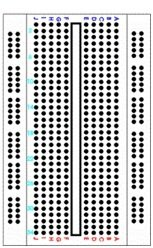Figure 1: BreadBoard

Figure 2a shows the manner in which the inner perforations are connected. Each row of 5 holes is “shorted”, that is, each hole in a row is connected to each other through an internal wire. The large rectangle in the center represents a grove. It should be noted here that holes on one side of the grove are not connected to the holes on the other side. Figure 2b shows the connections of the outer perforations. The holes are shorted column wise with no connections between the adjacent columns. Also, while the number of perforations in a column may vary with the length of the breadboard, there is always a break in connection at the half way point in a column as shown in the figure. This means that each column is essentially split into two smaller columns.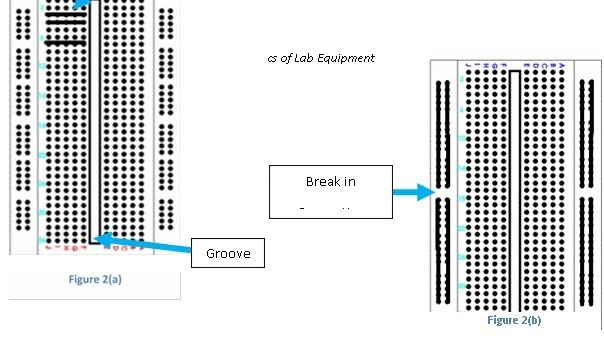### 2.  Power Supply for ICs

In electronics lab components, the components that you will be dealing with mostly are Integrated Circuits (ICs). There are several different types of ICs, each with its unique functionality. Figure 3 shows the structure of a typical IC chip package (called IC for convenience).  Every IC has a grove at the top which  is used to determine its orientation. Figure 4 shows the manner in which an IC is placed on the breadboard. It is recommended to ensure that the groove on the IC is pointing towards the left as illustrated in the figure. ICs are active elements. This means that they require to be “powered up” before   they   can   be   used.   Therefore,   every   IC,   no   matter   what its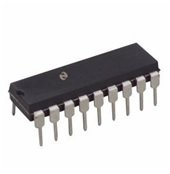Figure 3

functionality, will always have two special pins labeled Vcc and Ground (or Gnd). Ground is to be connected  to  the  Gnd  supply  of  the  trainer  board while Vcc is to be connected to the 5V supply. Ignore the 12V and the -12V supplies on the board.It should be noted here that voltage is a relative quantity. The voltage at any point can only be expressed as the amount of difference between that point   and   the  voltage   at   a   reference  point. The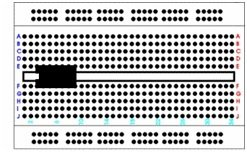Figure 4

reference point that you will be using is called Ground. Ground is all the connections on the trainer board that are assumed to be at 0V and all voltages marked on the trainer board are with reference to Ground pins. For example, the pin marked +5V is at a voltage that is 5 Volts greater than the Ground pin. This also means that if the pin marked +5V is assumed to be at 0V, then the Ground pin will be at -5V. When using multiple trainer boards, make sure that the ground pin of the trainer boards are shorted to ensure that the reference voltage is the same throughout the circuit.

### 3.  Logic Switches

Logic switches are the basic buildig blocks of electronics lab components. The inputs to a logic circuit are composed of a collection of ON/OFF signals. An ON signal (or logic ‘1’) is generated by applying +5V to an input pin while an OFF signal (or logic ‘0’) is generated   by applying 0V to an input pin. Figure 5 shows the internal structure of a switch in the ON and OFF state. When the switch is closed (by moving the switch down), it outputs +5V while when  the switch is opened (by moving it up), the output of the same pin is now 0V. This   is   called   “Positive   Logic   Connection”.   To   provide  these ON/OFF signals, the trainer boards are equipped with the following logic switches

#### 1.       Inverting switches (set A/set B)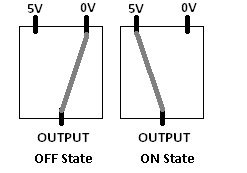Figure 5

These switches have 2 outputs. The first output is the normal output, which follows the convention described above. The second (inverted) output is the inverse of the first output, that is, if the normal output is 5V, then the inverted output is 0V and vice versa. The inverted output is typically denoted with an apostrophe (‘).

#### 2.       Non Inverting Switches

These switches have a single output, which follows the normal convention as stated above.

#### 3.       Debouncing Switches

When a normal switch is “thrown”, that is its state is changed, the resulting output voltage does not immediately go to and stay at the new value. Rather, it oscillates between 5V and 0V for a short period before settling at desired output. In sensitive circuits, this will lead to the circuit producing incorrect results. A debouncing switch employs a circuit to remove these oscillations and produce a clean transition when the switch is thrown.

### 4.  LED output

The outputs of logic circuits, like the inputs, are composed of ON/OFF signals. They can be verified by using  the  LEDs available  on  the  trainer  boards.  LED  stands  for Light  Emitting Diode;  a  two terminal device only conducts in one direction, as is the case with a typical diode. They convert electrical energy into light energy. The conversion results in a drop in voltage across the terminals of the LED, which is typically around 2V. LEDs offer a big advantage over traditional bulbs in that they consume negligible amount of power. The disadvantage of LEDs is their inability to withstand high voltages or currents. Therefore, when using an LED, always connect a resistor (100-200Ω) in series with it.

The LEDs present in the trainer board already have one terminal connected to the ground and resistor connected to the other terminal internally. Therefore, you do NOT need to connect a resistor when  using the LEDs on the trainer board. To test an output of an IC, connect it directly to the input terminal of an LED made available on the trainer board. If an LED turns on, then the output state at that pin is ON (+5V). If it remains off, then the pin is in OFF state (0V). Remember to turn on the trainer board before using the LEDS (in case you are using an external supply to power up your circuit).

### 5.  Seven Segmented Display

The seven-segmented display allows outputs to be viewed in decimal form. It takes 4 inputs (binary)  and, depending on their state, turns on LEDs in the display to show the corresponding digit.  For example, if the input to the display is 0111, then the display will show the number 7. If the input to the display is a number >9 (1001 in binary), the display will not show a number. Instead, it will display a unique character corresponding to that binary input. To turn off the display, apply the input 1111 to it.

### 6.  Clock

The trainer board provides a square wave that oscillates between a minima of 0V and a maxima of 5V. This is called a clock. The clock is used to drive “sequential” logic devices, which you will come across in the later labs. Essentially, this clock is used for the same purpose as the clock in you computers. The trainer board provides the option of varying the frequency of   this clock, but the amplitude is fixed.

### The Power Supply

Sometimes in electronics lab components, you may need to supply voltages other than those available on the trainer boards. For this purpose, you will be using  a power supply (Figure 6). The power supplies present in the lab are able to provide 2 independent variable voltages ranging from 0V to up to 30V and a fixed voltage supply of 5V. Each voltage output block consists of positive terminal (red) and a negative terminal (black). In some cases, a third terminal labeled Gnd (yellow/green) may be present but this can be ignored. The voltage being supplied by the power supply is essentially  the voltage of the positive terminal with respect to the negative terminal. To generate positive voltages, the negative terminal is shorted with the ground of the circuit and voltage is obtained from the positive terminal.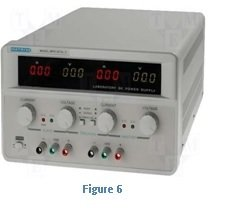To generate negative voltages, the positive terminal is shorted with the ground of the circuit and voltage is obtained from the negative terminal. The display will always indicate a positive value but the actual sign of the output voltage depends on the manner in which the terminals are connected. Note that, in using multiple voltage outputs from a single supply or from multiple power supplies, all the terminals taken to be at 0V should be shorted together.

Voltage can be varied on the variable outputs of the supply by rotating the respective voltage knobs. Apart from the voltage knob, there is one for current as well. The purpose of this knob is not to generate current as current depends entirely on the voltage being supplied and the power being drawn by the circuit. This knob is used to limit current, that is, it controls the maximum amount of current that can flow from the output block. If current being drawn from the supply tries to increase beyond the limit,  the red indicator LED turns on and the voltage being supplied drops even though the voltage knob is still in the same position. Since logic circuits draw a very small amount of current, it is a good idea to keep the limit to a small value.

### The Digital Multi Meter (DMM)

The multimeter (Figure 7), as suggested by its name, is used to measure multiple parameters in a circuit. It can be used to measure voltage, current, resistance, capacitance and it  even has a mode for checking for short circuits. In logic circuits, you will typically be using only the voltage and the short circuit mode.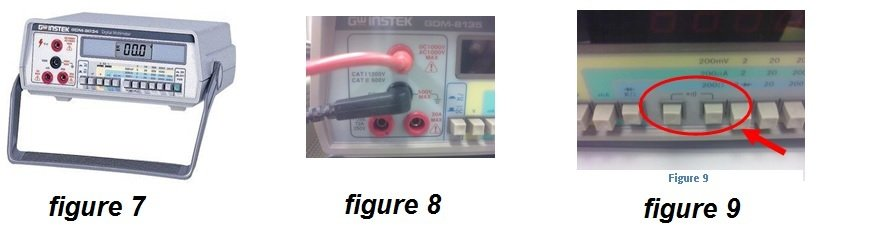#### 1.       Voltage

The voltage mode is accessed by pressing the button marked

“V”. Similar to the principle of generation, voltages are measured as the voltage at the red probe with respect to the black probe. The probes are connected to the multimeter as shown in Figure 8. In most cases, the black probe is shorted with the ground of the circuit. However, for cases such as measuring the potential drop across a resistor, the probes may be placed at the terminals of the resistor while it is inside the breadboard. The value of potential drop being displayed may either be positive or negative and this can be used to determine the direction in which current  is  flowing in the resistor.

#### 2.       Short Circuit

The short circuit mode is accessed by pressing the three buttons marked in Figure 9. All three buttons should be in a pushed in state. In this mode, the two probes can be used to check if a short circuit exists between two points in a circuit. The mode works  by  checking  if  a  closed  loop  is  formed  between the probes. A closed loop is a path in a circuit is one in which current can flow. This means that if two points are shorted together, current will flow between them. If there are not connected, then there is no  closed path for the current to travel (open loop) and hence no current will flow.

### The Oscilloscope

The oscilloscope (Figure 10) is one of the most interesting pieces of equipment that you will be using in the lab. Although it can only be used to measure voltages, it allows for a large amount of functions to be performed on the acquired data. It  is particularly helpful in measuring voltages that change with time as it is able to not only plot the wave form but also automatically measure many parameters associated with the wave. The probes of an oscilloscope are similar to the one shown in Figure 11. The small black clip is connected to the ground of the circuit whereas the larger grey part of the probe is connected to the point whose voltage needs to be  measured.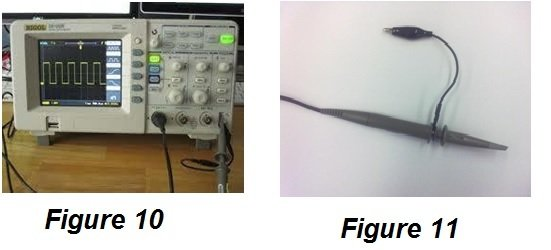In  electronic lab, the DMM is used to measure voltage whereas the oscilloscope is used to observe signal wave forms.

## The Function Generator electronics lab components

The function generator (Figure 12) is used to produce wave forms of variable frequency, amplitude and offset. The generators in the lab are capable of producing sine waves, triangle/saw-tooth waves and square waves. The principle of voltage generation is again similar to the power supply in which the negative (black) clip is connected to the ground and the voltage at the positive (red) clip oscillates with respect  to  it.  While  you  may  be  familiar  with  the  terms frequency and amplitude, offset is a relatively new yet equally important quantity.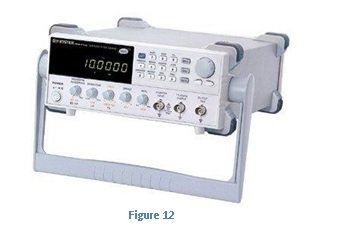Without offset, a generated waveform oscillates about the 0V line with the maximum and minimum voltages being A and –A (A = amplitude). This means that for half the time period, the output voltage is positive and for the remaining part, the output voltage is negative. If an offset of, for example, A volts is added, the maximum and minimum voltages become 2A and 0 respectively, thus ensuring the output voltage is always positive. For an offset of –A, the output voltage is always negative. Any value of offset will thus result in a change in the maximum and minimum values of output voltage. Two observations should be made here. Firstly, an offset does not change the shape of the waveform, since the voltage at each point in the wave is changed by the same relative amount. Secondly, in the case of a square wave,  if magnitude of the offset is < A, then the wave is still positive and negative for the same period of time as would have been for a zero offset.